# High School Chemistry : Help with Enthalpy

## Example Questions

### Example Question #1 : Help With Enthalpy

Consider the following balanced reaction: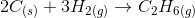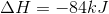What is the change in enthalpy if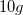of solid carbon is used in the above reaction?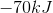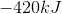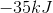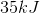Explanation:

The enthalpy of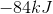describes the amount of heat when the amount of carbon in the balanced reaction (two moles) is used. Since onlyof carbon are used, we can find how much heat is released.

When two moles of carbon are used,are released. Two moles of carbon is equal to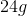of carbon, based on carbon's atomic mass.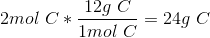Knowing this, we can set up proportions in order to determine how much heat is released byof carbon.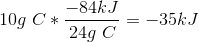Soof carbon results inof heat being released to the surroundings.

### Example Question #11 : Thermochemistry And Energetics

Consider these two half reactions:

Step 1.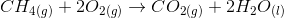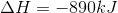Step 2.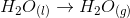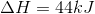Based on these half reactions, find the enthalpy for the following reaction: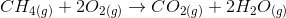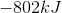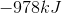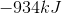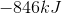Explanation:

Hess's law states that the enthalpy of the total reaction is equal to the enthalpy of the steps required to get to the total reaction, regardless of the path that is chosen. This means that we can combine the two half steps with known enthalpies in order to solve for the enthalpy of the main reaction.

Step 1.Step 2.Total:Since step 1 results in two moles of liquid water, we need to use the second step twice in order to replace them with two moles of water vapor.

Combined: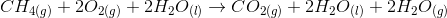Since the total reaction is created by step 1 occurring once and step 2 occurring twice, we can write the enthalpy as: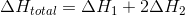Use the given enthalpies of the steps to calculate the total change in enthalpy.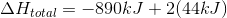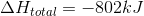### Example Question #1 : Help With Enthalpy

How much energy is required to heat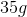of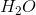from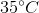to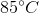?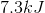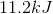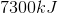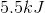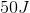Explanation:

Use the following formula: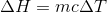Plug in values: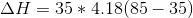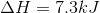### All High School Chemistry Resources